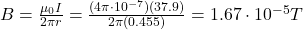## A 37.9 A current flows in a long, straight wire. Find the strength of the resulting magnetic field at a distance of 45.5 cm from the w

Question

A 37.9 A current flows in a long, straight wire. Find the strength of the resulting magnetic field at a distance of 45.5 cm from
the wire.

in progress 0
3 weeks 2021-09-03T17:56:42+00:00 1 Answers 0 views 0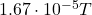Explanation:

The magnitude of the magnetic field produced by a current-carrying wire is given by the equation: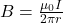where: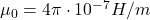is the vaacuum permeability

I is the current in the wire

r is the distance from the wire

The direction of the magnetic field lines is tangential to concentric circles around the wire.

In this problem, we have: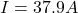is the current in the wire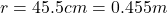is the distance  from the wire

Solving for B, we find the magnitude of the magnetic field: